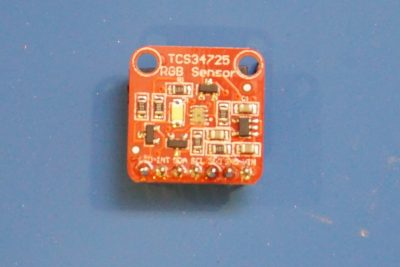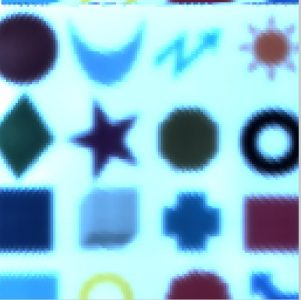A flat bed scanner typically uses three rows of CCD sensors (RGB) to capture images placed directly on top of the glass bed. When the CCD array scans from one end of the image to the other, the digitized color image is formed. So with a similar approach, we could use just one photosensitive device to capture the entire image one pixel at a time via raster scanning. Now that I have an HP 7044A X-Y recorder I could use it’s X/Y servo mechanism with a suitable sensor to build a single pixel scanner.

The simplest sensor would be just a photodiode or a CdS photocell. For capturing grayscale images, either of these sensors could be used. To capture color images though, we would need a sensor that is capable of discerning the RGB components of each pixel. For that I used an inexpensive TCS34725 based color sensor module.I mounted the color sensor onto the pen holder of the plotter and adjusted the height so that when the pen is down the sensor is roughly 4 to 5 mm above the surface. This should allow the obtained image to be more focused as less stray light would fall onto the sensor at any given pixel position. Ideally though I could have mounted a lens in front of the color sensor, but I did not have any suitable lens for this purpose.

The following pictures illustrate the experiment setup. I used an Arduino Due to control the plotter. Arduino Due is very convenient as it has two 12 bit DAC to control the X and Y movements of the plotter.

For the code below, I used Adafruit’s TCS34725 library for Arduino. One problem of the TCS34725 sensor is that for higher color resolution the capture time is rather long due to the long integration time. This unfortunately slows down the image scanning quite a bit. I could use a shorter integration time but the color resolution would be greatly reduced. So in the sample code below I used an image size of just 128×128 with a step size of 32 and used a relatively long integration time. Even at this resolution (128×128) it still takes close to an hour to finish scanning a single image. It would take more than a month to scan the same image at full resolution (4096×4096), which is impractical.

The captured RGB values at each pixel location is spit out to the serial console and are saved into a text file.

```#include <Wire.h>

int imageSize = 128;
int stepSize = 4096 / imageSize;

void setup(void) {
Serial.begin(9600);
analogWriteResolution(12);

delay(500);

if (tcs.begin()) {
Serial.println("Start>>");
runScan();
} else {
Serial.println("TCS34725 not detected");
}
}

void runScan()
{
uint16_t r,g,b,c;

int x,y;

for (x=0; x< 4096; x+=stepSize) {
analogWrite(DAC0,x);
delay(500);
for (y=0; y< 4096; y+=stepSize) {
analogWrite(DAC1,y);
delay(10);
tcs.getRawData(&r, &g, &b, &c);
Serial.print(r);Serial.print(", ");
Serial.print(g);Serial.print(", ");
Serial.print(b);Serial.print(", ");
}
Serial.println();
}
}

void loop(void) {
}
```

The following MATLAB code reads in the data captured in the text file, separates out the RGB components into their corresponding matrices and finally combines the three color channels back into a single color image.

```ftoread = 'img.txt';
M = textscan(fid, '%f', 'Delimiter',',');
fclose(fid);

rawImg = M{1};

r1 = (rawImg - min(rawImg))./max(rawImg)*3;

R=r1(1:3:length(r1));
G=r1(2:3:length(r1));
B=r1(3:3:length(r1));
r=reshape(R, [128, 128]);
g=reshape(G, [128, 128]);
b=reshape(B, [128, 128]);

rgbImg=cat(3, r, g, b);
imshow(rgbImg);
```

Here is the output from one of the experiment runs. As you can see, despite the low resolution all features in the original image are correctly captured although there is some color accuracy issues with the scanned image. The inaccuracies in the reproduced colors could be attributed to the sensor itself and the spectrum limitations of the onboard white LED.The short video below shows this scanner in action.